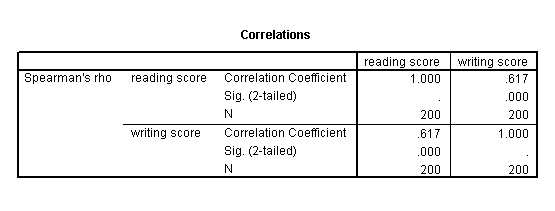## Non-parametric correlation

A Spearman correlation is used when one or both of the variables are not assumed to be normally distributed and interval (but are assumed to be ordinal). The values of the variables are converted in ranks and then correlated. In our example, we will look for a relationship between read and write. We will not assume that both of these variables are normal and interval.

nonpar corr /variables = read write /print = spearman.The results suggest that the relationship between read and write (rho = 0.617, p = 0.000) is statistically significant.